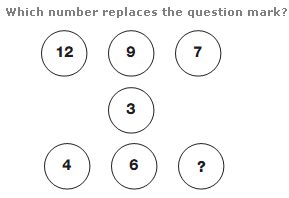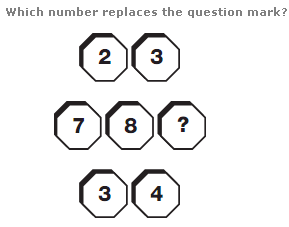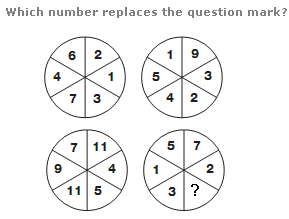# Puzzles - Number puzzles

Exercise : Number puzzles
94.:
9
Explanation
:
Starting with the numbers in the top row, and following straight lines through the centre of the diagram, subtract 3 from the number and put the result in the corresponding lower circle.

95.:
2
Explanation
:
Reading the top and bottom lines as 2 digit numbers, multiply these together to give the 3 digit result, written in the middle.

96.